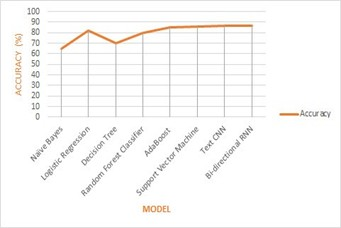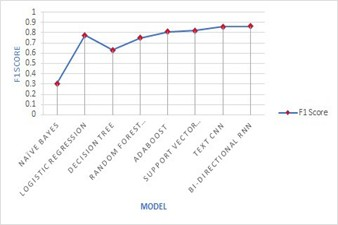## Insincere Questions Classification - Comparative study of different classification algorithms

Quora is a question-and-answer platform where questions are asked, answered, edited, and organized by community of users in the form of opinions . A key challenge for Quora is to remove insincere questions and only allow to have sincere questions to enter the system. Different Machine learning classification algorithms are used and compared for the classification. The data set provided by Quora is obviously comprised of textual data hence proper pre-processing of the input data is required to enhance the performance of the ML classification models. I have used word2vec model bag-of-words as an input and have done comparative study on different ML classification algorithms with state of the art neural nets that is graphical models.

I.INTRODUCTION
Website like Quora has toxic content and it is a quite huge problem. The work from this project is going to identify whether questions asked on Quora are sincere or not using various classification approaches. In the training data label column from training data represents whether the question is sincere or not. As the dataset is comprised of textual data, feature extraction from that textual data was the key challenge for us. Initially, I implemented most popular feature extraction model for text data:”Latent Dirichlet Allocation(LDA)” , ”Phraseminer”,Bag-of-words, using word count vectorizer as well as tf-idf(Term Frequency inverse Document Frequency) vectorizer. The important challenge of using Bag-of-Words model is that,with large datasets, the features extracted also grow rapidly,resulting in the curse of dimensionality.and it needs to be executed on the given system configurations.Using word2vec model, each word can be represented into a vector of integers and used as input for machine learning models. Word2vec model resolves curse of dimensionality and helped us with uniform features extraction giving better performance. The embedded data is then used on various models: Logistic Regression, Decision Tree, Random Forest,Naive Bayes, Support Vector Machines, Ada Boost and Neural Networks. The article includes detailed analysis and comparison of these models. Comparison is based on F1 score which is a good metric for imbalanced data. Removing skewness from the given dataset is important. Among 8 implemented models Text Convolutional Neural Network(CNN) and Bidirectional RNN that is graphical models performed better than traditional models as Text-CNN considers words in close range for context while Bidirectional RNN considers sequence and closeness of words for context.

II.DATA ANALYSIS

Overview:
Quora provided a good amount of training and test datato identify the insincere questions. Train data consists of 1.3 million rows and 3 columns in each row. Percentage of Sincere questions is 93.82% and percentage of insincere questions is 6.18%.
Data fields:
•qid: Unique question identifier
•question: Quora question’s text
•target: A question labeled insincere has a value of 1 otherwise 0
In the input data distribution of target variable i.e. sincere and insincere questions is an important measure. It gives basic idea about the data. As we can see from the data distribution table, data is highly imbalanced. Most of the Machine Learning classification models only performs optimally when the number of samples of each class is roughly the same. Classification of highly skewed datasets, where the minority is heavily outnumbered by one or more classes is a challenge. Re-sampling of the dataset is one of the possible solution, as to offset this imbalance with the hope of arriving at a more robust and fair decision boundary than you would otherwise. ”Input decides the quality of output” is the thumb rule in the field of machine learning. pre-processing is the next important step while dealing with textual dataset. I used below pre-processing steps:
•Removed the hyperlinks from the questions if present.
•Applied contractions (convert don ́t to do not)
•Spelling corrections. (becme to become)
•Removed everything except for words like numbers,special character(@,#,\$).
•Converted the input data into lower case.
•Removed short words like a, the etc.
•Performed stemming and lemmatization on data.

III.METHODOLOGY

A.Data Preparation

•Bag of words model
– Term frequency- Inverse document frequency
A numerical statistic reflects importance of a word to a document (question in our case) in the given data corpus. After pre-processing of the input data, a Q*V sparse matrix is created where rows represent a question and column represents tf-idf  smoothed frequency of words.
– Count vectorizer
A numerical statistic taking fre-quency of all the words in a question into consideration to make the input feature vector. This creates asparse Q*V matrix where a row represent a question and the column represents count of word.
•Word2Vec model
In Word2Vec model each word is represented by a point in the embedding space and these points are learned and moved around based on the words that surround the target word. Embedding helps us to learn the semantics of a sentence.We have used multiple embeddings as below.
– Google Embedding:Google embedding includesword vectors for a vocabulary of 3 million wordsand phrases that they trained on roughly 100 billionwords from a Google News dataset.
– Customized Embedding:We have created embeddings using Word2Vec algorithm on our training dataset

IV.IMPLEMENTATION

The entire implementation can be done using Python as a programming language and different machine learning libraries such as sklearn, nltk etc . As mentioned above I experimented multiple feature extraction methods like ”bag-of-words model”, (tf-idf model and word countvectorizer), ”word2vec model”. After doing research I found that Google embeddings performed better than other implemented methods. So feature extraction was done using google embedding method. Out of these google embedding vectors are then used as an input for the Machine learning classification algorithms. After doing initial data preprocessing I found that the maximum length of question allowed on Quora platform is 72 words in length. Using Google embeddings, I converted each word into a vector of 300 integers, each word vector appended next to each other. For instances where the question length was lesser than 72 words, we appended zeros to the final vector of that question, which created uniform feature set of dimension 21,600. There are options to reduce this dimension using different techniques like averaging vectors and min or max vectors. But this would loose the semantics  and correlation between data
and will add noise to it. To avoid this I did not reduce the dimesnion further.
Our dataset is huge, so balanced datasets are generated using hybrid sampling (SMOTE + ENN)  with sincere to insincere ratio of 3:2 and 20,000 data samples. After trying different combination and research work the classification models are trained with below parameters for classification.

 Model Parameter Decision tree criterion = ’gini’, min samples split = 2 Random Forest n estimators =10, min samples split =2 Logistic Regression intercept scaling =1 , max iter =100 SVM kernel = rbf, Degree = 3, Penalty = 1 Text CNN activation Conv2D =elu, output=sigmoid Bi-directional RNN activation Middle = relu, output=sigmoid Ada-boost iterations =1000, classifier = LR

TABLE : Parameters of Model

Sklearn split method was used to divide the training and validation dataset. I decide to divide the input training data into train and validation set in 9:1 ratio. I compared the performance of these models by considering Accuracy and also F-1 scores.

V. RESULTS

Out of 8 different Machine learning models used, I found that the Bi-directional RNN performed better in terms of accuracy as Ill as F1 scores, followed by Text CNN and Support Vector Machine. Naive Bayes tradition ML algorithm was the least performing algorithm as expected (As Naive Bayes considers each feature as independent from others, this assumption does not hold true in case of textual data as words in sentence are related to each other.)Fig 1. compares Accuracy VS Model and Fig 2. compares F1 Score VS Model.
Support Vector Machine  [SVM] performed better among the traditional models as it implementations the data in the higher dimensions using rbf kernel,which helps to find the optimal decision boundary. SVM is more robust due to optimal margin gap between separating hyper planes, it could do better predictions with test data . In Text CNN, result of each convolution will fire when a special pattern is detected. By varying the size of the kernels and concatenating their outputs, we allow to detect patterns of multiples sizes. CNNs can identify patterns in the sentence regardless of their position . This pattern identification from words in close range improved performance.
In Bi-directional RNN , It is stated that the sequence of words which are close to each other. This sequence identification from words in close range improved performance even better than Text CNN.Fig. 2: F1 Score Results

REFERENCES

 Wikipedia contributors, “Quora — Wikipedia, the free encyclopedia,”https://en.wikipedia.org/w/index.php? title=Quoraoldid=893103406,2019, [Online; accessed 22-April-2019].

 W. Li, L. Sun, and D.-K. Zhang, “Text classification based on labeled-lda model,”CHINESE JOURNAL OF
COMPUTERS-CHINESE EDITION-,vol. 31, no. 4, p. 620, 2008.

 A. El-Kishky, Y. Song, C. Wang, C. R. Voss, and J. Han, “Scalable topical phrase mining from text
corpora,”Proceedings of the VLDB Endowment, vol. 8, no. 3, pp. 305–316, 2014.

 C.-H. Huang, J. Yin, and F. Hou, “A text similarity measurement combining word semantic information with tf idf method,”Jisuanji Xuebao(Chinese Journal of Computers), vol. 34, no. 5, pp. 856–864,2011.

 H. T. Madabushi and M. Lee, “High accuracy rule-based question classification using question syntax and semantics,” in Proceedings of COLING 2016, the 26th International Conference on Computational Linguistics: Technical Papers, 2016, pp. 1220–1230.

 G. E. Batista, R. C. Prati, and M. C. Monard, “A study of the behavior of several methods for balancing
machine learning training data,”ACMSIG KDD explorations newsletter, vol. 6, no. 1, pp. 20–29, 2004.

 J. Xu, Y. Zhou, and Y. Wang, “A classification of questions using svm and semantic similarity analysis,” in 2012 Sixth International Conference on Internet Computing for Science and Engineering. IEEE, 2012, pp.31–34.

 F. Colas and P. Brazdil, “Comparison of svm and some older classification algorithms in text classification tasks,” in IFIP International Conference on Artificial Intelligence in Theory and Practice. Springer,2006, pp. 169– 178.

 A. Jacovi, O. S. Shalom, and Y. Goldberg, “Understanding convolutional neural networks for text
classification,”arXiv preprint arXiv:1809.08037, 2018.

 P. Zhou, Z. Qi, S. Zheng, J. Xu, H. Bao, and B. Xu, “Text classification improved by integrating bidirectional lstm with two-dimensional maxpooling,”arXiv preprint arXiv:1611.06639, 2016

Search
Categories